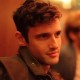# John

## 28 Jan 2013In my implementation of Textrain I read a string into an array of letter objects. Each object knows its character, xOffset, what column its in and where it is currently located vertically. Each object scans it’s column for the highest dark spot and then checks that against it’s current location. Thus, each object can make a determination about whether it should fall as normal or cling to the highest dark pixel.
A few details worth noting.

1. I flipped the pixel array so that the image is mirrored, this is nicer for display.
2. I added a meager easing function to ‘captured’ letters to help keep them from wiggling
3. I basically ignore the bottom 50 pixels due to vignetting.

Textrain from john gruen on Vimeo.

Code is available at Github:https://github.com/johngruen/Textrain

```import processing.video.*;
Capture v;
int totalOffset = 0; //helper var for counting xOffset for each Letter obj
PImage flip; //buffer for horizontally flipped image
String fallingLetters = "Lorem ipsum dolor sit amet, consectetur adipiscing elit. Etiam non aliquam ante. Nullam at ligula mi. Nam orci metus";
Letter[] letters;
PFont font;
int fontSize = 32;
float thresh = 100; // brightness threshold, Use UP and DOWN to alter
float sampleFloor = 40; //stop sampling pixels 20 rows from the bottom
color activeColor = color (255,0,255);
color fallColor = color(20,100);

void setup() {
size(1280,720);
v = new Capture(this,width,height,30);
flip = createImage(width,height,RGB);
textFont(font,fontSize);
initLetters();
}

void draw() {
flipImage(); //flip the image
v.updatePixels();
image(flip,0,0);
for(int i = 0; i < letters.length; i++) {
letters[i].draw();
}
flip.updatePixels();

}

void initLetters() {
letters = new Letter[fallingLetters.length()];
for(int i = 0; i < letters.length; i++) {
letters[i] = new Letter(i,totalOffset,fallingLetters.charAt(i));
totalOffset+= textWidth(fallingLetters.charAt(i));
}
}

void flipImage() {
for(int y = 0; y < v.height; y++) {
for(int x = 0; x < v.width; x++) {
int i = y*v.width + x;
int j = y*v.width + v.width-1-x;
flip.pixels[j] = v.pixels[i];
}
}
}

void keyPressed() {
if (keyCode == UP) thresh++;
else if (keyCode == DOWN) thresh--;
println(int(thresh));
}

class Letter {
int index; //just a good thing to know, used for debugging
int xOffset; //offset horizontally of letter registration
float speed; //speed
char c; //what letter am i
float curYPos, prevYPos; //current and previous y position. used for easing.
int state = 0; // we use a tiny state switcher to control the flow. either the letter is falling or it isn't
float topBrightPixel;//get top bright pixel;

Letter(int index_,int xOffset_,char c_) {
index = index_;
xOffset = xOffset_;
c = c_;
curYPos = int(random(-200,-100));//set currentYPos somewhere above the video
speed = int(random(5,12));
}

void draw() {
senseBrightness();
compareToCurrent();
update();
text(c,xOffset,curYPos);
}

void senseBrightness() {
topBrightPixel = 0;
for(int i = xOffset; i < flip.pixels.length-flip.width*sampleFloor; i+=flip.width) {
if(brightness(flip.pixels[i]) < thresh) {
break;
}
topBrightPixel++;
}
}

void compareToCurrent() {
if (topBrightPixel > curYPos + 2*speed || topBrightPixel >= flip.height - sampleFloor) {
state = 0;
//speed = random(5,12);
} else {
state = 1;
}
}

void update() {
switch (state) {
case 0:
fill (fallColor);
curYPos+=speed;
speed = speed * 1.02;
if (curYPos > height + 50)  {
curYPos = random(-50,-200);
speed = random(5,12);
}
break;
case 1:
fill(activeColor);
curYPos += .6* (topBrightPixel - prevYPos);
speed = random(5,12);
break;
}
prevYPos = curYPos;
}

}
```